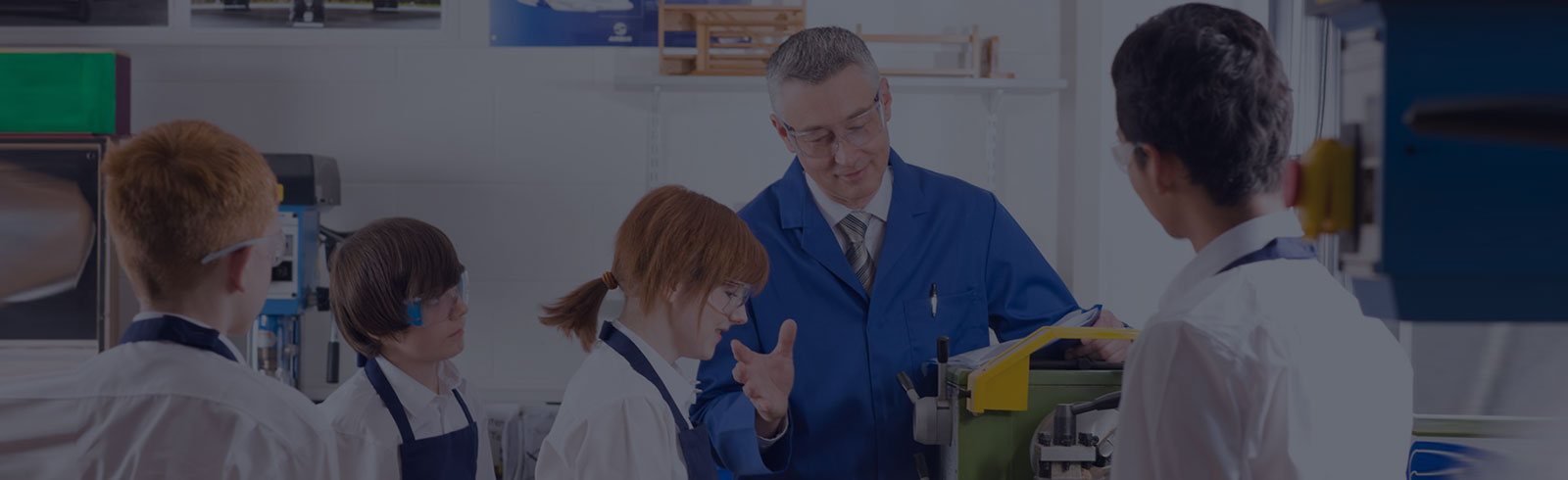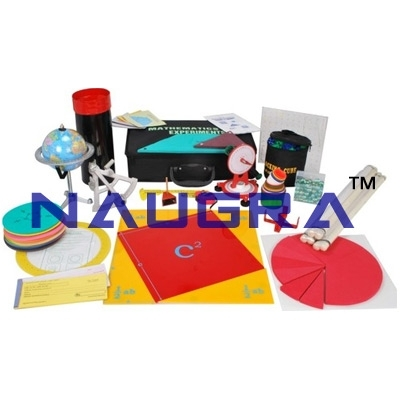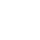Products
Categories

# Mathematics Lab KitNaugra Export is a leading Mathematics lab kit manufacturers, suppliers and exporters company in India. Our wide range of mathematics laboratory kits are tested on various parameters to ensure their adherence with international standards.

We are supplying high-quality Mathematics lab kit to the various schools, colleges, universities, research labs and educational institutes around the world at the most affordable prices.

Some of our highly demanded Mathematical laboratory kits are Junior Mathematics Kit, Senior Mathematics Kit, Square Set, Tangram, Dice, Stencil, Circle, Square & Geometric Stencil, Protractor, Test Board, Number Balance, Centimeter of Cubes etc.

### Junior Mathematics Kit for Maths Lab

Lab Supply Code: MATHS-KIT001

### Senior Mathematics Kit for Maths Lab

Lab Supply Code: MATHS-KIT002

### Square Set for Maths Lab

Lab Supply Code: MATHSKITS003

### Tangram for Maths Lab

Lab Supply Code: MATHSKITS004

### Dice for Maths Lab

Lab Supply Code: MATHSKITS005

### Color Dice for Maths Lab

Lab Supply Code: MATHSKITS006

### Stencil for Maths Lab

Lab Supply Code: MATHSKITS007

### Geometric Stencil for Maths Lab

Lab Supply Code: MATHSKITS008

### Stencil for Maths Lab

Lab Supply Code: MATHSKITS009

### Circles, Squares, Geometric Stencil for Maths Lab

Lab Supply Code: MATHSKITS0010

### Stencil Lettering 15mm, for Maths Lab

Lab Supply Code: MATHSKITS0011

### Count and See for Maths Lab

Lab Supply Code: MATHSKITS0012

### Protractor for Maths Lab

Lab Supply Code: MATHSKITS0013

### Test board 2pcs/set for Maths Lab

Lab Supply Code: MATHSKITS0014

### Centimetre of cubes for Maths Lab

Lab Supply Code: MATHSKITS0015

### Centimetre of cubes for Maths Lab

Lab Supply Code: MATHSKITS0016

### Multibase for Maths Lab

Lab Supply Code: MATHSKITS0017

### Multibase for Maths Lab

Lab Supply Code: MATHSKITS0018

### Multibase for Maths Lab

Lab Supply Code: MATHSKITS0019

### Multibase for Maths Lab

Lab Supply Code: MATHSKITS0020

### Number balance for Maths Lab

Lab Supply Code: MATHSKITS0021

### Combination Bucket for Maths Lab

Lab Supply Code: MATHSKITS0022

### QHS-008 for Maths Lab

Lab Supply Code: MATHSKITS0023

### Elementary two-pan balance for Maths Lab

Lab Supply Code: MATHSKITS0024

### Plastic two-bucket balance for Maths Lab

Lab Supply Code: MATHSKITS0025

### Wood scale for Maths Lab

Lab Supply Code: MATHSKITS0026

### Counting Stick for Maths Lab

Lab Supply Code: MATHSKITS0027

### Counting Stick for Maths Lab

Lab Supply Code: MATHSKITS0028

### Counting Stick for Maths Lab

Lab Supply Code: MATHSKITS0029

### Geometric pyramid for Maths Lab

Lab Supply Code: MATHSKITS0030

### Teacher and Pupil Clock for Maths Lab

Lab Supply Code: MATHSKITS0031

### Geometry board for Maths Lab

Lab Supply Code: MATHSKITS0032

### Rulers for Maths Lab

Lab Supply Code: MATHSKITS0033

### Geometry board for Maths Lab

Lab Supply Code: MATHSKITS0034

### Geometry board for Maths Lab

Lab Supply Code: MATHSKITS0035

### Geometry board for Maths Lab

Lab Supply Code: MATHSKITS0036

### Round Fraction set for Maths Lab

Lab Supply Code: MATHSKITS0037

### Round Fraction set for Maths Lab

Lab Supply Code: MATHSKITS0038

### Round Fraction set for Maths Lab

Lab Supply Code: MATHSKITS0039

### Fraction set for Maths Lab

Lab Supply Code: MATHSKITS0040

### Fraction set for Maths Lab

Lab Supply Code: MATHSKITS0041

### Fraction demonstration board for Maths Lab

Lab Supply Code: MATHSKITS0042

### Geometrical feature for Maths Lab

Lab Supply Code: MATHSKITS0043

### Geometrical feature for Maths Lab

Lab Supply Code: MATHSKITS0044

### Geometrical feature for Maths Lab

Lab Supply Code: MATHSKITS0045

### Geometrical feature for Maths Lab

Lab Supply Code: MATHSKITS0046

### Geometrical feature for Maths Lab

Lab Supply Code: MATHSKITS0047

### Geometrical feature (dissectable) for Maths Lab

Lab Supply Code: MATHSKITS0048

### Geometrical feature for Maths Lab

Lab Supply Code: MATHSKITS0049

### Geometrical feature for Maths Lab

Lab Supply Code: MATHSKITS0050

### Letter Card for Maths Lab

Lab Supply Code: MATHSKITS0051

### Count Card for Maths Lab

Lab Supply Code: MATHSKITS0052

### Fold Rule(200cm) for Maths Lab

Lab Supply Code: MATHSKITS0053

### Count piece with two color for Maths Lab

Lab Supply Code: MATHSKITS0054

### Graph block for Maths Lab

Lab Supply Code: MATHSKITS0055

### Geometry physique for child for Maths Lab

Lab Supply Code: MATHSKITS0056

### Splicing bards for Maths Lab

Lab Supply Code: MATHSKITS0057

### Turnplate of color lump for Maths Lab

Lab Supply Code: MATHSKITS0058

### Pattern blocks for Maths Lab

Lab Supply Code: MATHSKITS0059

### Calender stamp for Maths Lab

Lab Supply Code: MATHSKITS0060

### Pattern blocks for Maths Lab

Lab Supply Code: MATHSKITS0061

### Plastic counter for Maths Lab

Lab Supply Code: MATHSKITS0062

### T square for Maths Lab

Lab Supply Code: MATHSKITS0063

### Color Ball for Maths Lab

Lab Supply Code: MATHSKITS0064

### Plastic counter for Maths Lab

Lab Supply Code: MATHSKITS0065

### Cube set for Maths Lab

Lab Supply Code: MATHSKITS0066

### Geometric shapes set for Maths Lab

Lab Supply Code: MATHSKITS0067

### Intelligence toy for Maths Lab

Lab Supply Code: MATHSKITS0068

### Counter for Maths Lab

Lab Supply Code: MATHSKITS0069

### Logic diagram Set for Maths Lab

Lab Supply Code: MATHSKITS0070

### Multibase for Maths Lab

Lab Supply Code: MATHSKITS0071

### Student desks and chairs for Maths Lab

Lab Supply Code: MATHSKITS0072

### Sliding board for children for Maths Lab

Lab Supply Code: MATHSKITS0073

### Sliding board for children for Maths Lab

Lab Supply Code: MATHSKITS0074

### Sliding board for children for Maths Lab

Lab Supply Code: MATHSKITS0075

### Wood balance for Maths Lab

Lab Supply Code: MATHSKITS0026